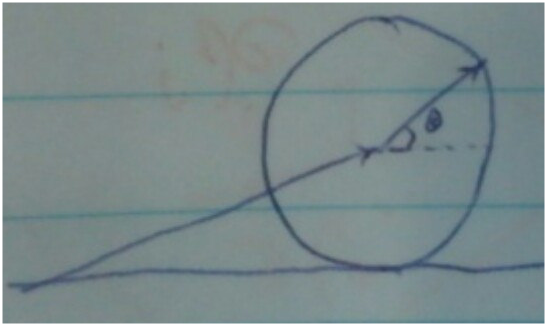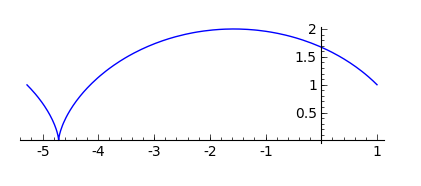## Shape of cycloid and its parametric equation:

Shape of cycloid and its parametric equation:

Take derivative at $\theta = 0$ and you will find (using l'Hopital's rule) $\frac{dx/d\theta}{dy/d\theta} = \infty$. i.e. the curve is going straight up at the origin!

You can also see it as a sum of two vectors:Notice here the circle is rolling from right to left, so the formula is a bit different.

\begin{align*} \begin{pmatrix} -rt \\ r \end{pmatrix} + \begin{pmatrix} r\cos t \\ r\sin t \\ \end{pmatrix} \end{align*}

Plot in sage:Double click to toggle code
sage: t = var('t')
sage: parametric_plot( (cos(t) - t, sin(t) + 1), (t, 0, 6.28), plot_points=200 )